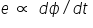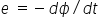Faraday connected a coil to a galvanometer and brought a magnet close to the coil or took it away form the coil. In both the case he observed deflection in the galvanometer. In his experiment he moved relative to the coil in such a way that magnetic flux linked with the coil due to which current is produced so the galvanometer shows the deflection. this phenomenon due to which a EMF is induced due to  varying  magnetic flux in known as Electromagnetic Induction.

Based upon this experiment he gave 3 laws known as Faraday’s laws of electromagnetic induction.

The varying flux would produce an EMF in the wire.

1. 1st Law :- whenever these in a varying magnetic flux associated with a conductor and EMF is induced in it.

If the varying flux is associated with a closed conductor like loop of closed circuit etc then due to the induced EMF the current shall start flowing in the closed circuit known as induced current. Further due to the induced current magnetic field is produced known as induced magnetic field.

1. 2nd Law :- the induced EMF tool as long as flux remain varying.

The moment flux become constant then irrespective of it’s magnitude induced EMF disappears.

1. 3rd Law :- the magnitude of induced EMF varies directly with rate of change of magnetic flux.d φ =varying M. flux

e = EMF (volts)Volt can also be expressed as Weber/sec

Significance of –ve sign :-

-ve sign indicates that the direction of induced EMF is such that it opposes the change in flux

EMF in induced due to the change in flux. Flux can charge in two weep. It can either grow as our decay. If flux is growing then EMF is induced in such a way that magnetic field induced by it is directed opposite to the applied field is opposed. On the other hand if the applied flux is decaying then induced field the same direction. As that of the opposed field such that it supports the applied field so an to support the decay. In both the cases the direction of induced flux is such that it opposes change in the applied flux.

Lenz’s law :-

It states that the direction of induced EMF is such that it opposes every that cause which produces it.

Lenz’s law and conservation of energy

Considered north pole of a magnet moving close to loop. The flux linked to the loop would increase. EMF should be induced in such a way which induces magnetic field in a direction that the face of the loop behaves as north pole which would repel the north pole of the magnet due to the Lenz’s law. If we wish move the magnet closer to the loop against the repulsive force we must exert internal force on the magnet as a result we shall perform mechanical work which appears as elective of energy in the loop.

Lenz’s law is in accordance  with law of conservation of energy.

Related Keywords